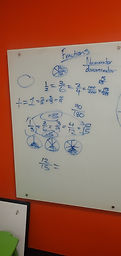## Mr. Jake

### Target 1​

###### Lesson Type:

Continuation

Number Operation

:

Fractional Numbers

Identity, compare, and manipulate different types of fractions.

###### 1:

Understand that fractions represent quantities that have a portion that is less than a whole.

###### 2:

Understand that fractions with different numerators and denominators can represent the same size.

###### 3:

Simplify a fraction to its lowest terms.

###### 4:

Create equivalent fractions with common denominators.

###### 5:

Use understandings of factors to simplify fractions.

5th

###### Vocabulary:

Fractions, Denominator, Numerator, LCM

Activities:

Practiced Adding and subtracting fractions with unlike denominators

Determined if fractions are equivalent

Learned how LCM can be used to add fractions with unlike denominators### Home Exploration

###### Guiding Questions:## Absent Students:

### Target 2

:

###### Vocabulary:

Activities:### Home Exploration

###### Guiding Questions:### Target 3

:

###### Vocabulary:

Activities:### Home Exploration